Home

# A NOTE ON THE DIOPHANTINE EQUATION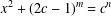$x^{2}+(2c-1)^{m}=c^{n}$

## Abstract

Let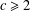$c\geq 2$ be a positive integer. Terai [‘A note on the Diophantine equation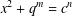$x^{2}+q^{m}=c^{n}$ ’, Bull. Aust. Math. Soc.90 (2014), 20–27] conjectured that the exponential Diophantine equation$x^{2}+(2c-1)^{m}=c^{n}$ has only the positive integer solution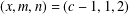$(x,m,n)=(c-1,1,2)$ . He proved his conjecture under various conditions on$c$ and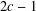$2c-1$ . In this paper, we prove Terai’s conjecture under a wider range of conditions on$c$ and$2c-1$ . In particular, we show that the conjecture is true if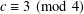$c\equiv 3\hspace{0.6em}({\rm mod}\hspace{0.2em}4)$ and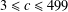$3\leq c\leq 499$ .

## Footnotes

Hide All

This research was supported by the National Natural Science Foundation of China (grant no. 11601108) and the Natural Science Foundation of Hainan Province (grant no. 20161002).

## References

Hide All
MathJax
MathJax is a JavaScript display engine for mathematics. For more information see http://www.mathjax.org.

# A NOTE ON THE DIOPHANTINE EQUATION$x^{2}+(2c-1)^{m}=c^{n}$

## Metrics

### Full text viewsFull text views reflects the number of PDF downloads, PDFs sent to Google Drive, Dropbox and Kindle and HTML full text views.

Total number of HTML views: 0
Total number of PDF views: 0 *Loading metrics...

### Abstract viewsAbstract views reflect the number of visits to the article landing page.

Total abstract views: 0 *Loading metrics...

* Views captured on Cambridge Core between <date>. This data will be updated every 24 hours.

Usage data cannot currently be displayed